# AP Chemistry : Oxidation State

## Example Questions

### Example Question #1 : Oxidation State

When is the oxidation number of H (-1)?

Only when it is bonded to Chlorine
When it is bonded to a more electronegative element
When it is bonded to a less electronegative element
Never
Only when it is bonded to Flourine
Correct answer: When it is bonded to a less electronegative element
Explanation:

We typically think of Hydrogen as having an oxidation number of +1.  However when it is bonded to a less electronegative element such as Na it is actually assigned an oxidation number of -1.

### Example Question #2 : Oxidation State

What is the oxidation number of manganese in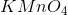?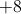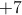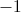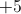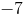Explanation:Potassium always has an oxidation state of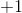, while oxygen always has an oxidation state of. Since we have four oxygens, there is a total charge offrom them.

The most important rule of oxidation numbers is that their sum must equal the charge on the molecule. In this compound, we have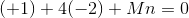.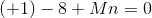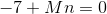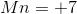Manganese needs to cancel out the charge from potassium and oxygen in order to give us a neutral compound.

### Example Question #3 : Oxidation State

What is the oxidation number of chlorine in perchlorate?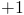Explanation:

Perchlorate is a complex ion with the formula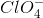.

The most important rule for oxidation number is that the sum of the oxidation states of the atoms must equal the overall molecular charge.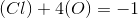This compound has four oxygens, which always have aoxidation state.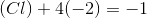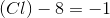Chlorine usually has an oxidation state of, but in this case it must balance out the oxygens.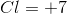Chlorine must be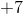.

### Example Question #4 : Oxidation State

Consider the following balanced equation: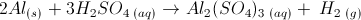What is the difference between the oxidation state of aluminum on the right side of the equation versus the left?No differenceExplanation:On the left side of the equation,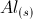is a solid, so its oxidation state is zero, but on the right side it is in a salt, so it is not in its zero state.

Sulfate,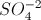, is an anionic salt, and there are three sulfate ions in each complex, yielding a net charge of -6. The two aluminum ions must have a net charge of +6, which, divided over two aluminum ions, yields an oxidation state of +3 for each aluminum ion.

The difference comes from simple subtraction: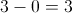.

### Example Question #5 : Oxidation State

What is the oxidation number of Cr, S, and Fe in the following substances: (a) K2Cr2O7 (b) H2SO4 (c) Fe2O3.

6, 6, 3

3, 6, 3

3, 6, 6

6, 6, 6

3, 3, 3

6, 6, 3

Explanation:

(a) Since O has a –2 oxidation number and K has a +1 oxidation number (1 valence
electron it gives up), that means that Cr must have an oxidation number of +6. (b) Since H
has a +1 oxidation number and O has a –2 oxidation number, S has a +6 oxidation number.
(c) Fe has an oxidation number of +3 in order for it to have a net 0 oxidation state.

### Example Question #6 : Oxidation State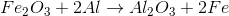In the above reaction, what are the initial and final oxidation states of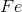?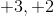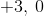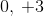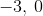There is no change in oxidation stateExplanation:

To determine the initial oxidation state of, we first must realize that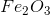has 3 oxygen atoms, each with a charge of, for a total charge contribution of. Furthermore, sincehas no net charge, theatoms must contribute a total charge of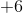to balance out thecharge coming from the oxygens. And since there are twoatoms, then each must have a charge of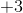.

On the product side of the reaction, notice thatis all by itself without any charge. The oxidation state of any individual atom is.

### Example Question #7 : Oxidation State

The Claus process is used in the petroleum industry to convert sulfur containing gases into solid (rhombic) sulfur. One of the reactions that takes place in this process is: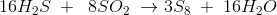Which of the following statements is correct:

All of them are correct

This is a red-ox reaction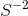gets oxidizes to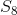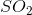is the oxidant

All of them are correct

Explanation:

We have an oxidizing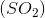and a reducing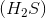agent, then is a red-ox reaction and the two semi reactions are: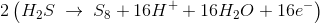(oxidation,losses electrons)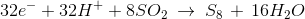(reduction,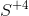gains electrons)

In total 32 electrons are transferred from theto.